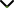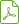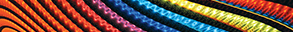Fractional and Time-Scales Differential Equations

Publishing date
27 Dec 2013
Status
Published
09 Aug 2013

Guest Editors

1Department of Mathematics and Computer Sciences, Cankaya University, Ankara, Turkey

2Department of Mathematics, Faculty of Science, King Abdulaziz University, Jeddah, Saudi Arabia

3Department of Mathematics, University of Aveiro, Aveiro, Portugal

4Department of Mathematics, Mobarakeh Branch, IAU, Iran

Fractional and Time-Scales Differential Equations

DescriptionThe theory and applications of fractional differential equations are gaining relevance since they are used in the modeling of different processes in physics, chemistry, and engineering.

Time-scale formalism unifies the theories of difference and differential equations. Therefore, time-scale analysis constitutes a good tool to study both discrete and continuous systems.

Recently, several attempts have been done to join the two subjects, developing a fractional calculus on time scales. The subject is still much evolving, and contributions joining the two areas are particularly welcome.

In order to apply fractional and/or time-scale differential equations for solving real problems, we need to add some uncertainty in the modeling. Therefore, often, problems are set-valued, for example, interval, fuzzy, or stochastic problems. Sometimes, combinations can be applied for different types of fractional and/or time-scale differentiability.

The special issue is focused on latest results in fractional and/or time-scale differential equations and their applications. Potential topics include, but are not limited to:

• Set-valued ordinary and partial differential equations
• Set-valued equations on time scales
• Fractional set-valued differential equations
• Equations with impulses
• Calculus of variations and optimal control with time-scale and/or fractional derivatives
• Interval and fuzzy fractional/time-scale differential equations
• Stochastic set-valued differential systems with error analysis
• Existence, uniqueness, and stability of solutions
• Numerical simulations and computational aspects
• Fractional impulsive differential equations with uncertainty
• Fractional/time-scale numerical methods with uncertainty
• Local fractional operators
• Applications of fractional and/or time-scale calculus
• Applications to real world problems

Before submission authors should carefully read over the journal’s Author Guidelines, which are located at http://www.hindawi.com/journals/aaa/guidelines/. Prospective authors should submit an electronic copy of their complete manuscript through the journal Manuscript Tracking System at http://mts.hindawi.com/submit/journals/aaa/frats/ according to the following timetable:Download Special IssueJournal metrics
Acceptance rate14%
Submission to final decision40 days
Acceptance to publication54 days
CiteScore1.200
Journal Citation Indicator-
Impact Factor-Author guidelinesEditorial boardDatabases and indexing

Article of the Year Award: Outstanding research contributions of 2020, as selected by our Chief Editors. Read the winning articles.